# PSEB 4th Class Maths Solutions Chapter 3 Fractional Numbers Ex 3.1

Punjab State Board PSEB 4th Class Maths Book Solutions Chapter 3 Fractional Numbers Ex 3.1 Textbook Exercise Questions and Answers.

## PSEB Solutions for Class 4 Maths Chapter 3 Fractional Numbers Ex 3.1

Question 1.
Match the fraction according to coloured portion: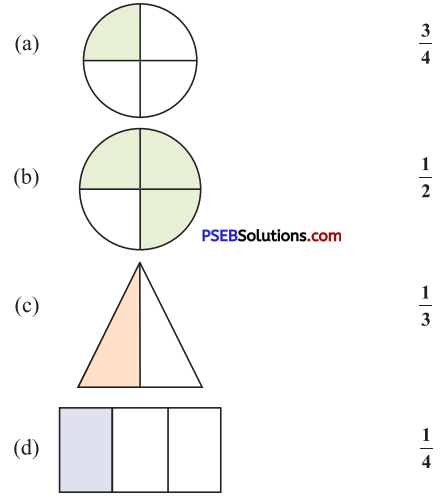Solution:
(a) $$\frac{1}{4}$$
(b) $$\frac{3}{4}$$
(c) $$\frac{1}{2}$$
(d) $$\frac{1}{3}$$Question 2.
Write the fraction of coloured as well as blank portion in the given space :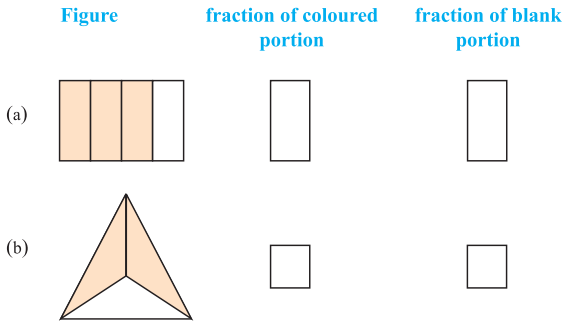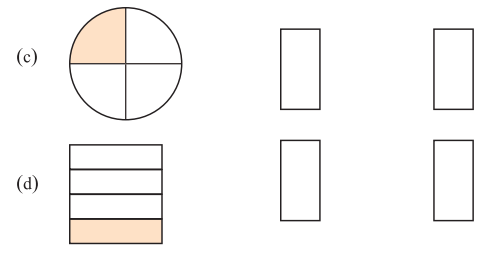Solution: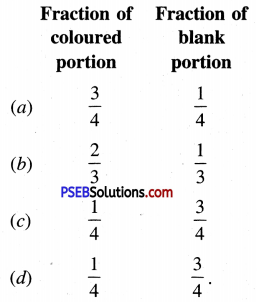3. Colour the figure according to the given fraction :

Question 1.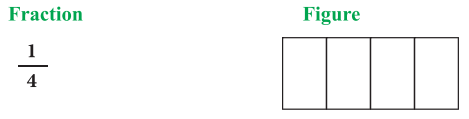Solution: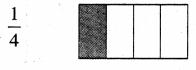Question 2.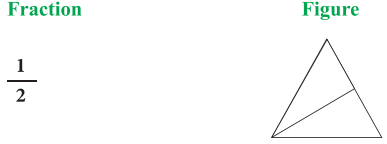Solution: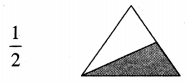Question 3.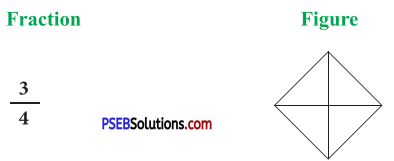Solution: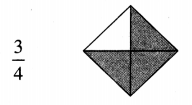Question 4.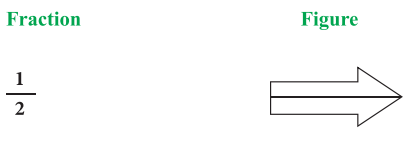Solution: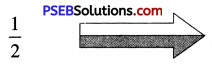Question 5.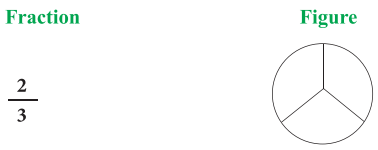Solution: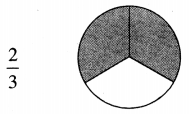Question 6.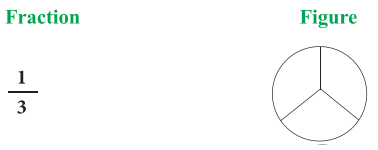Solution: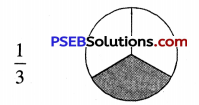4. Mark (✓) on the correct fraction of the coloured portion of the figure:

Question 1.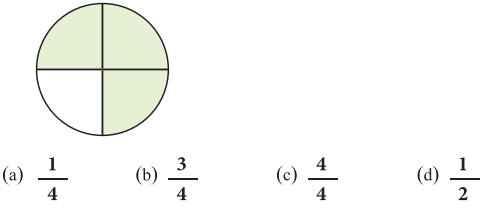Solution:
(b) $$\frac{3}{4}$$Question 2.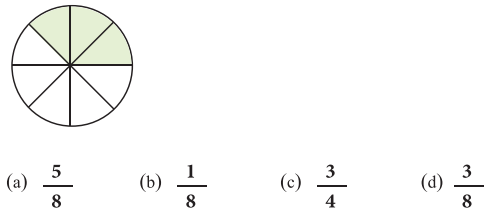Solution:
(d) $$\frac{3}{8}$$

Question 3.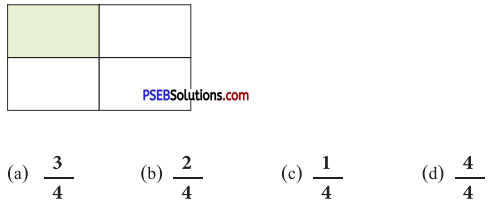Solution:
(c) $$\frac{1}{4}$$

5. Write the given fraction in words:

Question 1.
$$\frac{1}{2}$$
Solution:
Half

Question 2.
$$\frac{1}{4}$$
Solution:
One Fourth

Question 3.
$$\frac{1}{3}$$
Solution:
One thirdQuestion 4.
$$\frac{2}{3}$$
Solution:
Two third

Question 5.
$$\frac{3}{4}$$
Solution:
Three fourth

Question 6.
$$\frac{1}{10}$$
Solution:
One tenth.

6. Write numerator and denominator of the given fractions :

Question 1.
$$\frac{2}{3}$$
Solution:
Numerator = 2 and Denominator = 3

Question 2.
$$\frac{1}{2}$$
Solution:
Numerator = 1 and Denominator = 2Question 3.
$$\frac{1}{4}$$
Solution:
Numerator = 1 and Denominator = 4

Question 4.
$$\frac{3}{4}$$
Solution:
Numerator = 3 and Denominator = 4.